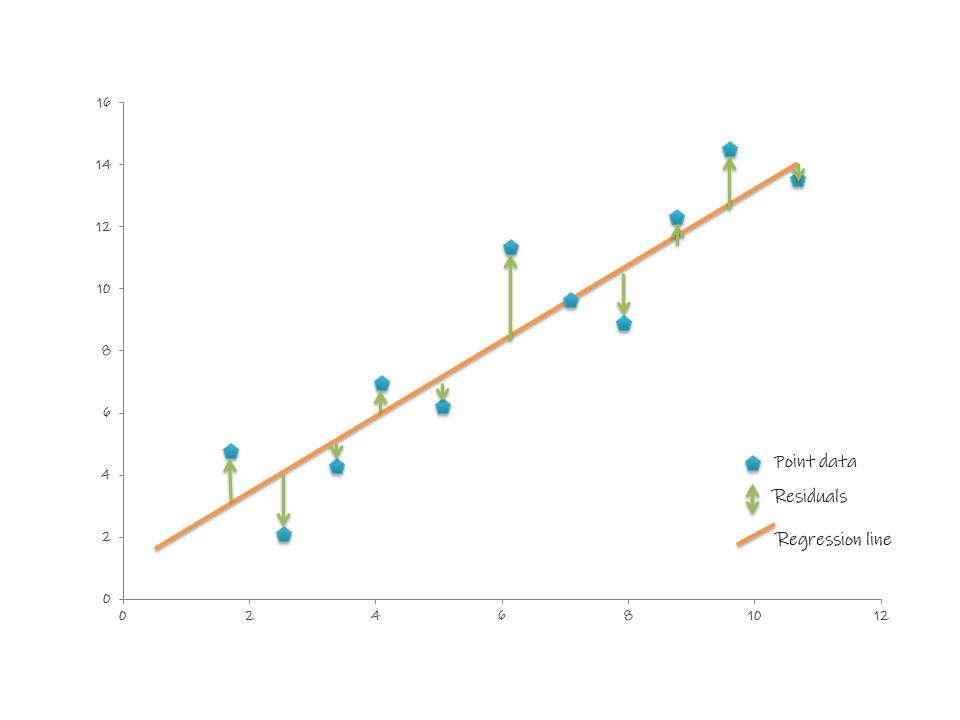## O#

### One-hot encoding#

One-hot encoding is a way to represent categorical variables as numerical data, so that it can be used in machine learning algorithm. It involves creating a new binary column for each unique category in the categorical feature.

For example, if a categorical feature has three categories, A, B, and C. Then three new columns, one for each category would be created. If a given data point belongs to category A, then the value in the A column would be 1, and the values in the B and C columns would be 0.

IDNameCategoryCategory ACategory BCategory C
1AppleA100
2BananaB010
3CherryC001

One-hot encoding allows the model to treat each category as a separate entity, rather as a numeric value that can be compared or ordered.

## R#

### Root Mean Square Error (RMSE)#

Root Mean Square Error (RMSE) is the standard deviation of the residuals (prediction errors). Residuals are a measure of how far from the regression line data point are. RMSE is a measure of how spread out these residuals are. In other word, it tells you how concentrated the data is around the line of best fit. Root mean square error is commonly used in climatology, forecasting, and regression analysis to verify experimental results.

$$RMSE = \frac{1}{2}$$